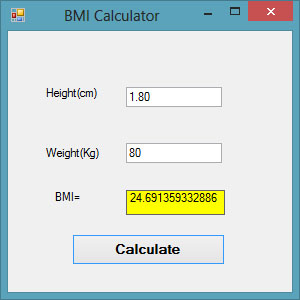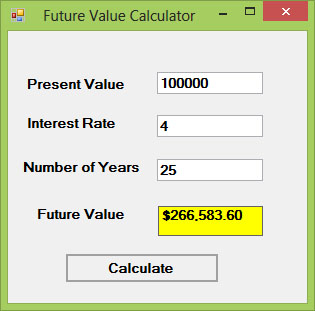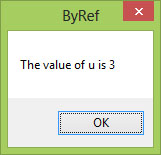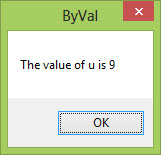# Visual Basic 2013 Lesson 17: Creating a Function

### 17.1 Creating User-Defined Functions

A function is similar to a sub procedure,  both are called by the main procedure to fulfil certain tasks. However, there is one difference, a function returns a value whilst a sub procedure does not. It merely performs a certain job. There are two types of functions, the built-in functions, and the user-defined functions.

To create a user-defined-function in Visual Basic 2013, you can type the function procedure directly into the code window as follows:

```Public Function functionName (Argument As dataType,..........) As dataType
```

or

`Private Function functionName (Argument As dataType,..........) As dataType`

*The keyword Public indicates that the function is applicable to the whole project and the keyword Private indicates that the function is only applicable to a certain module or procedure. An argument is a parameter that can pass a value back to the function. You can include as many arguments as you can.

#### Example 17.1: BMI Calculator

This BMI calculator is a Visual Basic 2013 program that can calculate the body mass index or BMI of a person based on the body weight in kilogram and the body height in meter. BMI can be calculated using the formula weight/( height )2, where weight is measured in kg and height in meter. If you only know your weight and height in lb and feet, then you need to convert them to the metric system.

If your BMI is more than 30, you are considered obese. You can refer to the following range of BMI values for your weight status.

• Underweight = <18.5
• Normal weight = 18.5-24.9
• Overweight = 25-29.9
• Obesity = BMI of 30 or greater

#### The Code

```Public Class Form1
Private Function BMI(Height As Single, weight As Single) As Double
BMI = weight / Height ^ 2
End Function

Private Sub BtnCal_Click(sender As Object, e As EventArgs) Handles BtnCal.Click
Dim h As Single, w As Single
h = Val(TextBox1.Text)
w = Val(TextBox2.Text)
LblBMI.Text = BMI(h, w)
End Sub
End Class
```

The outputFigure 17.1

#### Example 17.2: Future Value Calculator

The concept of future value is related to time value of money. For example, if you deposit your money in a bank as a savings account or a fixed deposit account for a certain period of time, you will earn a certain amount of money based on the compound interest computed periodically, and this amount is added to the principal if you continue to keep the money in the bank. Interest for the following period is now computed based on the initial principal plus the interest (the amount which becomes your new principal). Subsequent interests are computed in the same way.

For example, let’s say you deposited \$1000 in a bank and the bank is paying you 5% compound interest annually. After the first year, you will earn an interest of \$1000×0.05=\$50. Your new principal will be \$1000+\$1000×0.05=\$1000(1+0.05)=\$1000(1.05)=\$1050.

After the second year, your new principal is \$1000(1.05)x1.05=\$1000(1.05)2 =\$1102.50. This new principal is called the future value.

Following the above calculation, the future value after n years will be

`FV = PV * (1 + i / 100)n`

Where PV represents the present value, FV represents the future value, i is the interest rate and n is the number of periods (Normally months or years).

#### The Code

```Public Class Form1
Private Function FV(pv As Single, i As Single, n As Integer) As Double
FV = pv * (1 + i / 100) ^ n
End Function

Private Sub BtnCal_Click(sender As Object, e As EventArgs) Handles BtnCal.Click
Dim FutureVal As Single
Dim PresentVal As Single
Dim interest As Single
Dim period As Integer
PresentVal = TxtPV.Text
interest = TxtInt.Text
period = TxtN.Text
FutureVal = FV(PresentVal, interest, period)
LblFV.Text = Format(FutureVal, "\$#,##0.00")
End Sub
End Class
```

#### The OutputFigure 17.2

### 17.2 Passing Arguments by Value and by Reference

Functions can be called by value or called by reference.  By default, the arguments in the function are passed by reference. If arguments are passed by reference, original data will be modified and no longer preserved. On the one hand, if arguments are passed by value, original data will be preserved. The keyword to pass arguments by reference is ByRef and the keyword to pass arguments by value is ByVal.

For example,

`Private Function FV(ByVal pv As Single, ByRef i As Single, n As Integer) As Double`

The function FV receives pv by value, i by reference and n by reference. Notice that although ByRef is not used to pass n, by default it is passed by reference.

#### Example 17.2(a)

In this example, we created two functions that compute the square root of a number, the first uses the keyword ByRef and the second uses the keyword ByVal.

#### The Code

```Private Function sqroot(ByRef x As Single) As Double
x = x ^ 0.5
sqroot = x
End Function

Private Function sqroot1(ByVal y As Single) As Double
y = y ^ 0.5
sqroot1 = y
End Function

Private Sub Button1_Click(sender As Object, e As EventArgs) Handles Button1.Click
Dim u As Single
u = 9
MsgBox(3 * sqroot(u), , "ByRef")
MsgBox("Value of u is " & u, , "ByRef")
End Sub

Private Sub Button2_Click(sender As Object, e As EventArgs) Handles Button2.Click
Dim u As Single
u = 9
MsgBox(3 * sqroot1(u), , "ByVal")
MsgBox("Value of u is " & u, , "ByVal")
End Sub

```

#### The Output

Case 1: Passing argument using ByRefFigure 17.3

Notice that the value of u has been changed to 3

Case 2: Passing argument using ByValFigure 17.4

Notice that the value of u remains unchanged.